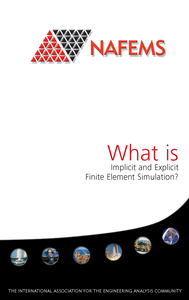## What is Implicit and Explicit Finite Element SimulationFInite element simulation is a numerical technique widely used to solve complex structural mechanics problems in engineering. The numerical solution can be achieved using either an implicit or explicit approach – the terms describing the way that the mathematical equations are formulated for solution. In engineering terms, the choice of implicit or explicit solution is generally determined by the nature of the engineering problem being solved and the important physics to be considered. In this short guide the different approaches and some typical applications are described, together with some important considerations for each.

### Document Details

Reference WT05 English Analyst Manager Publication 16th May 2014 NAFEMS Global